Equations > Calculus > Integration/Integrals

Showing results 1 to 9 of 9, on page 1 of 1
 Description Equation Integration by parts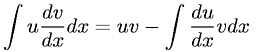Integral of powers not equal to -1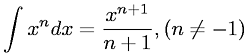Integral of reciprocal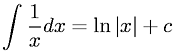Integral of cosine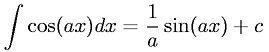Integral of sine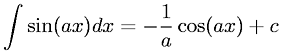Integral of tangent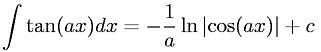Integral of cosecant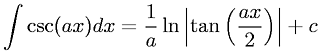Integral of secant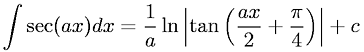Fundamental Theorem of Integrals of Derivatives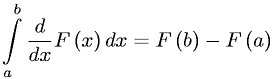(you need to login first)